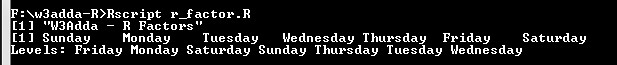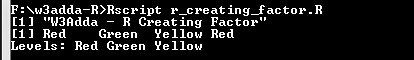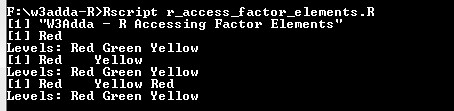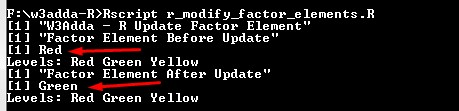# R Factors

In this tutorial you will learn about the R Factors and its application with practical example.

## R Factors

Factor is a data structure that can only contain predefined set of values(categorical data). Factors are useful when we want deal with limited set of values for variable, in this case we must define the possible values beforehand and all of the distinct values are termed as levels.

Example:-

Here, we have defined a factor that contains all of 7 weekdays as values.

Output:-

When we run the above R script, we see the following output –It list all of the factor values and levels. It has the 7 levels.

## Creating a factor

In R, a factor is created using factor() function. The factor() function takes a vector input in order to create a factor.

Syntax:-

Above is the general syntax of factor() function, here

data:- It is a vector input which contains all of the factor values.
levels:- It takes a vector input(lData) which contains all of the factor levels. Levels can be inferred from the data if not provided.

Example:-

Output:-## Accessing factor Elements

Factor elements can be accessed same way as of vectors, by passing index value(s) in brackets [ ] you can access factor elements. An index value can be logical, integer or character.

Example:-

Output:-## Modify Factor Element

A factor element can be modified by accessing element and assigning it new value from predefined set of values.

Example:-

Output:-Note:- If we try to assign values outside of its predefined levels, then it raise an error.

In this tutorial we have learn about the R Factors and its application with practical example. I hope you will like this tutorial.